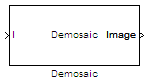# Demosaic

Demosaic Bayer's format images

## Library

Conversions

`visionconversions`

•## Description

The following figure illustrates a 4-by-4 image in Bayer's format with each pixel labeled R, G, or B.The Demosaic block takes in images in Bayer's format and outputs RGB images. The block performs this operation using a gradient-corrected linear interpolation algorithm or a bilinear interpolation algorithm.

PortInput/OutputSupported Data TypesComplex Values Supported

I

Matrix of intensity values

• If, for the Interpolation algorithm parameter, you select `Bilinear`, the number of rows and columns must be greater than or equal to 3.

• If, for the Interpolation algorithm parameter, you select `Gradient-corrected linear`, the number of rows and columns must be greater than or equal to 5.

• Double-precision floating point

• Single-precision floating point

• Fixed point

• 8-, 16-, and 32-bit signed integer

• 8-, 16-, and 32-bit unsigned integer

No

R, G, BMatrix that represents one plane of the input RGB video stream. Outputs from the R, G, or B ports have the same data type.Same as I port

No

Image

M-by-N matrix of intensity values or an M-by-N-by-P color video signal where P is the number of color planes.

Same as I port

No

Use the Interpolation algorithm parameter to specify the algorithm the block uses to calculate the missing color information. If you select `Bilinear`, the block spatially averages neighboring pixels to calculate the color information. If you select `Gradient-corrected linear`, the block uses a Weiner approach to minimize the mean-squared error in the interpolation. This method performs well on the edges of objects in the image. For more information, see .

Use the Sensor alignment parameter to specify the alignment of the input image. Select the sequence of R, G and B pixels that correspond to the 2-by-2 block of pixels in the top-left corner of the image. You specify the sequence in left-to-right, top-to-bottom order. For example, for the image at the beginning of this reference page, you would select `BGGR`.

Use the Output image signal parameter to specify how to output a color video signal. If you select `One multidimensional signal`, the block outputs an M-by-N-by-P color video signal, where P is the number of color planes, at one port. If you select `Separate color signals`, additional ports appear on the block. Each port outputs one M-by-N plane of an RGB video stream.

### Fixed-Point Data Types

The following diagram shows the data types used in the Demosaic block for fixed-point signals.You can set the product output and accumulator data types in the block mask as discussed in the next section.

## Parameters

Interpolation algorithm

Specify the algorithm the block uses to calculate the missing color information. Your choices are `Bilinear` or ```Gradient-corrected linear```.

Sensor alignment

Select the sequence of R, G and B pixels that correspond to the 2-by-2 block of pixels in the top left corner of the image. You specify the sequence in left-to-right, top-to-bottom order.

Output image signal

Specify how to output a color video signal. If you select ```One multidimensional signal```, the block outputs an M-by-N-by-P color video signal, where P is the number of color planes, at one port. If you select `Separate color signals`, additional ports appear on the block. Each port outputs one M-by-N plane of an RGB video stream.

Rounding mode

Select the rounding mode for fixed-point operations.

Overflow mode

Select the overflow mode for fixed-point operations.

Product outputAs depicted in the previous figure, the output of the multiplier is placed into the product output data type and scaling. Use this parameter to specify how to designate this product output word and fraction lengths:

When you select `Same as input`, these characteristics match those of the input to the block.

When you select `Binary point scaling`, you can enter the word length and the fraction length of the product output, in bits.

When you select `Slope and bias scaling`, you can enter the word length, in bits, and the slope of the product output. The bias of all signals in the Computer Vision Toolbox™ blocks is 0.

AccumulatorAs depicted in the previous figure, inputs to the accumulator are cast to the accumulator data type. The output of the adder remains in the accumulator data type as each element of the input is added to it. Use this parameter to specify how to designate this accumulator word and fraction lengths:

• When you select `Same as product output`, these characteristics match those of the product output.

• When you select `Same as input`, these characteristics match those of the input.

• When you select `Binary point scaling`, you can enter the word length and the fraction length of the accumulator, in bits.

• When you select `Slope and bias scaling`, you can enter the word length, in bits, and the slope of the accumulator. The bias of all signals in the Computer Vision Toolbox blocks is 0.

Lock data type settings against change by the fixed-point tools

Select this parameter to prevent the fixed-point tools from overriding the data types you specify on the block mask. For more information, see `fxptdlg` (Fixed-Point Designer), a reference page on the Fixed-Point Tool in the Simulink® documentation.

## References

 Malvar, Henrique S., Li-wei He, and Ross Cutler. “High-Quality Linear Interpolation for Demosaicing of Bayer-Patterned Color Images.” Microsoft Research, May 2004. http://research.microsoft.com/pubs/102068/Demosaicing_ICASSP04.pdf.

 Gunturk, Bahadir K., John Glotzbach, Yucel Altunbasak, Ronald W. Schafer, and Russel M. Mersereau, “Demosaicking: Color Filter Array Interpolation,” IEEE Signal Processing Magazine, Vol. 22, Number 1, January 2005.

## Version History

Introduced in R2006b